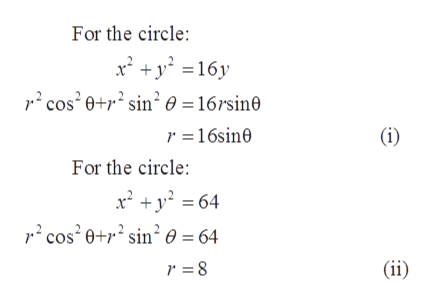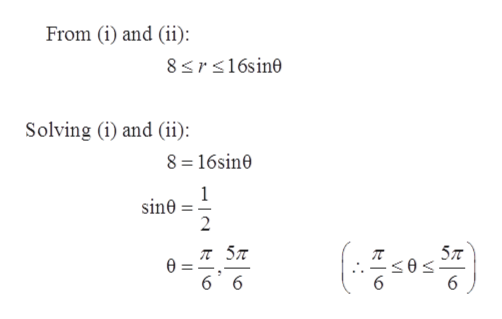# A lamina occupies the region inside the circle x^2+y^2=16 but outside the circle x^2+y^2=64 The density at each point is inversely proportional to its distance from the orgin.Where is the center of mass?

Question

A lamina occupies the region inside the circle x^2+y^2=16 but outside the circle x^2+y^2=64 The density at each point is inversely proportional to its distance from the orgin.

Where is the center of mass?

check_circleExpert Solution
Step 1

Given:

The density at each point is inversely proportional to its distance:

Step 2help_outlineImage TranscriptioncloseFor the circle: x2y16y cos20+2 sin 0= 16rsin0 (i) r 16sine For the circle: cos20+2 sin2 0 = 64 (ii) r 8 fullscreen
Step 3help_outlineImage TranscriptioncloseFrom (i) and (ii): 8srs16sine Solving (i) and (ii) 8 16sin0 1 sine 2 ( л 5л 5л 6 6 fullscreen

### Want to see the full answer?

See Solution

#### Want to see this answer and more?

Solutions are written by subject experts who are available 24/7. Questions are typically answered within 1 hour*

See Solution
*Response times may vary by subject and question
Tagged in

### Other